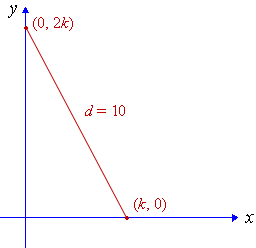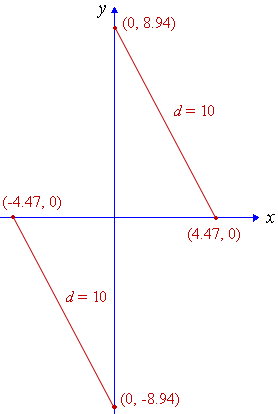Search IntMath
Close

450+ Math Lessons written by Math Professors and Teachers

5 Million+ Students Helped Each Year

1200+ Articles Written by Math Educators and Enthusiasts

Simplifying and Teaching Math for Over 23 Years

# 1. Distance Formula

We use the Distance Forumala to find the distance between any two points (x1,y1) and (x2,y2) on a cartesian plane.

### The Cartesian Plane

The cartesian plane was named after Rene Descartes.

See more about Descartes in Functions and Graphs.

Let's start with a right-angled triangle with hypotenuse length c, as shown:

Recall Pythagoras' Theorem, which tells us the length of the longest side (the hypotenuse) of a right triangle:

c=sqrt(a^2+b^2)

We use this to find the distance between any two points (x1, y1) and (x2, y2) on the cartesian (x-y) plane:

A (x1, y1)
B (x2, y1)
C (x2, y2)
distance = d

The point B (x2, y1) is at the right angle. We can see that:

• The distance between the points A(x1, y1) and B(x2, y1) is simply x2x1 and
• The distance between the points C(x2, y2) and B(x2, y1) is simply y2y1.
A (x1, y1)
B (x2, y1)
C (x2, y2)
x2 − x1
y2 − y1
distance = d

Distance from (x1, y1) to (x2, y2).

Using Pythagoras' Theorem we can develop a formula for the distance d.

## Distance Formula

The distance between (x1, y1) and (x2, y2) is given by:

d=sqrt((x_2-x_1)^2+(y_2-y_1)^2

Note: Don't worry about which point you choose for (x1, y1) (it can be the first or second point given), because the answer works out the same.

## Interactive Graph - Distance Formula

You can explore the concept of distance formula in the following interactive graph (it's not a fixed image).

Drag either point A (x1, y1) or point C (x2, y2) to investigate how the distance formula works. As you drag the points the graph will automatically calculate the distance.

Length AB = x2x1

Length BC = y2y1

Length

### Example 1

Find the distance between the points (3, −4) and (5, 7).

Here, x1 = 3 and y1 = −4; x2 = 5 and y2 = 7

So the distance is given by:

d=sqrt((x_2-x_1)^2+(y_2-y_1)^2)

=sqrt((5-3)^2+(7-(-4))^2)

=sqrt(4+121)

=11.18

### Example 2

Find the distance between the points (3, −1) and (−2, 5).

This time, x1 = 3 and y1 = −1; x2 = −2 and y2 = 5

So the distance is given by:

d=sqrt((x_2-x_1)^2+(y_2-y_1)^2)

=sqrt((-2-3)^2+(5-(-1))^2)

=sqrt(25+36)

=sqrt61

=7.8102

### Example 3

What is the distance between (−1, 3) and (−8, −4)?

In this example, x1 = −1 and y1 = 3; x2 = −8 and y2 = −4

So the distance is given by:

d=sqrt((x_2-x_1)^2+(y_2-y_1)^2)

=sqrt((-8-(-1))^2+(-4-3)^2)

=sqrt(49+49)

=sqrt98

=9.899

### Example 4

Find k if the distance between (k,0) and (0, 2k) is 10 units.

This is the situation:Applying the distance formula, we have:

d=sqrt((x_2-x_1)^2+(y_2-y_1)^2)

=sqrt((2k-0)^2+(0-k)^2)

=sqrt(4k^2+k^2)

=sqrt(5k^2)

Now sqrt(5k^2)=10 so 5k^2=100, giving:

k2 = 20

so

k=+-sqrt(20)~~+-4.472

We obtained 2 solutions, so there are 2 possible outcomes, as follows:## Problem SolverThis tool combines the power of mathematical computation engine that excels at solving mathematical formulas with the power of GPT large language models to parse and generate natural language. This creates math problem solver thats more accurate than ChatGPT, more flexible than a calculator, and faster answers than a human tutor. Learn More.